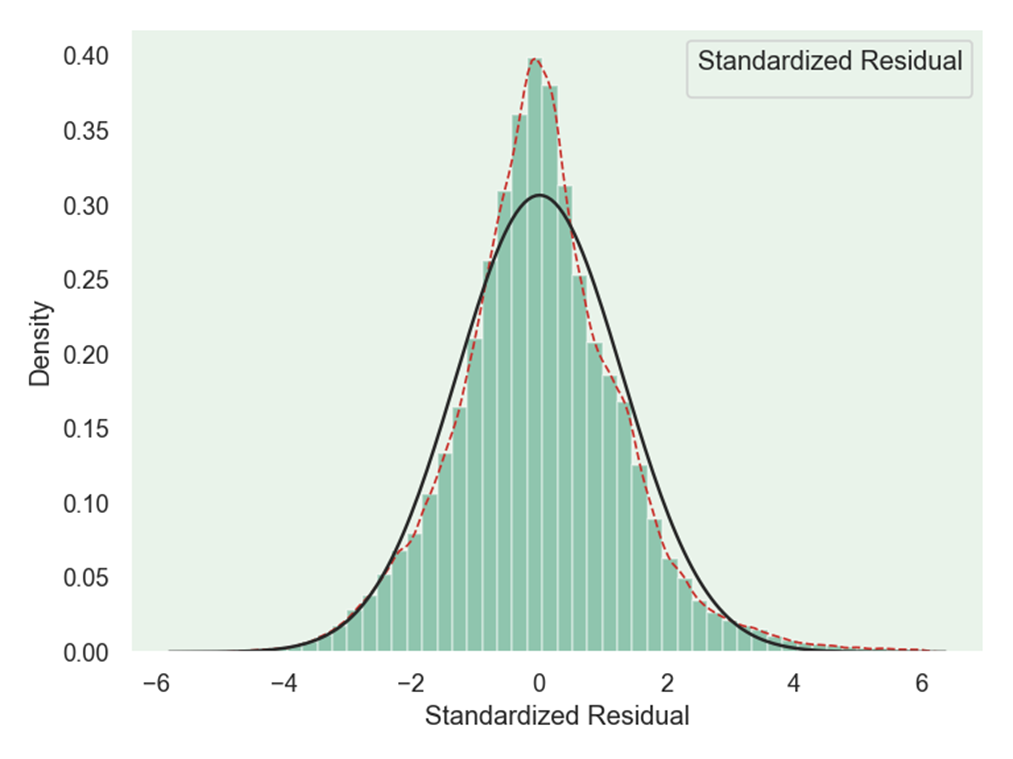• python做概率密度分布图
2021-12-04 09:32:29
for i in sorted(list(set(df['yearmonth']))):
df_sort = df[df['yearmonth'] == i].groupby('cust_id')['y','pred'].sum().sort_values('y',ascending = False)
df_sort['yearmonth'] = i
df_sort['y_cum'] = np.cumsum(df_sort['y'])
df_sort['y_cum_rate'] = df_sort['y_cum']/max(df_1_clear_sort['y_cum'])
df_sort['index'] = list(range(1,df_sort.shape+1))
df_sort['index_cum_rate'] = [i/df_sort.shape for i in list(range(1,df_sort.shape+1))]

plt.figure(figsize=(4,4))
plt.plot(list(df_sort['index_cum_rate']),list(df_sort['y_cum_rate']))
plt.title(i,fontsize=24)
plt.xlabel('index_cum_rate',fontsize=14)
plt.ylabel('y_cum_rate',fontsize=14)
plt.grid(True)
plt.show()

更多相关内容
• 目录   1、scipy库中各分布对应的方法   2、stats中各分布的常用方法及其功能   3、正态分布概率密度函数及其图象 ...    3）python绘制t分布和正态分布概率密度函数对比   6、F分布概率密度函数
• 主要介绍了python 计算概率密度、累计分布、逆函数的例子,具有很好的参考价值，希望对大家有所帮助。一起跟随小编过来看看吧
• 当我们想要弄清楚变量的统计特性时，往往想知道它是服从什么分布的，这时候就需要绘制概率分布直方 ...在seaborn中的distplot函数可以完成概率分布直方密度图的绘制 seaborn.distplot(a, bins=None, his.

当我们想要弄清楚变量的统计特性时，往往想知道它是服从什么分布的，这时候就需要绘制概率分布直方图

在python中我们可以使用seaborn库来进行绘制：

Seaborn是一个基于matplotlib的Python数据可视化库。它为绘制有吸引力和信息丰富的统计图形提供了高级界面。

首先需要导入seaborn库：

import seaborn as sns


在seaborn中的distplot函数可以完成概率分布直方图和密度图的绘制

seaborn.distplot(a, bins=None, hist=True, kde=True, rug=False,
fit=None, hist_kws=None, kde_kws=None, rug_kws=None, fit_kws=None,
color=None, vertical=False, norm_hist=False, axlabel=None,
label=None, ax=None)


下面直接给出我绘制时用到的代码：

import seaborn as sns
import matplotlib as mpl
import matplotlib.pyplot as plt
from scipy.stats import norm
from scipy.stats import laplace

txt=[]
data=[]
path = r"E:\rtklib\rtklib-test1\stav.txt"

#mpl.rc("figure", figsize=(9, 5))
with open(path, "r") as f:

for i in range(len(txt)):
data.append(float(txt[i].strip().split(',')))

sns.set_palette("hls")
#sns.set_style("whitegrid")
plt.figure(dpi=120)
sns.set(style='dark')
sns.set_style("dark", {"axes.facecolor": "#e9f3ea"})
g = sns.distplot(data,
hist=True,
kde=True,  # 开启核密度曲线kernel density estimate (KDE)
kde_kws={'linestyle': '--', 'linewidth': '1', 'color': '#c72e29',
# 设置外框线属性
},
fit=norm,
color='#098154',
axlabel='Standardized Residual',  # 设置x轴标题

)

plt.show()


其中，distplot的参数中，kde表示是否绘制核密度曲线；fit是选择拟合的分布，来分析数据究竟是符合什么分布，seaborn中提供了很多分布，可以在这里找到，使用时如上我的代码中导入的那样就可以：连续分布类型
最后的效果图如下：绘制过程中没有加入太多修饰，如果想要好看一点，可以修改一些参数：
其中一个有设置背景 的风格，主要有以下几种：

sns.set_style("whitegrid")
sns.set_style("dark")
sns.set_style("darkgrid")
sns.set_style("white")
sns.set_style("ticks")

展开全文算法
• 关于Python绘制正态分布图概率密度分布）以及遇到的问题（label无法显示） 目的：统计神经网络模型的参数分布情况 使用seaborn给我们提供的distplot函数来绘制，即调用sns.distplot()，并传入相关参数即可，这里...

# 关于Python绘制正态分布图（概率密度分布）以及遇到的问题（label无法显示）

目的：统计神经网络模型的参数分布情况

使用seaborn给我们提供的distplot函数来绘制，即调用sns.distplot()，并传入相关参数即可，这里我们用np.random.normal()函数来生成10000个均值为0，方差为1的数据，并将其传入sns.distplot()

from matplotlib import pyplot as plt
from scipy.stats import norm
import seaborn as sns
import numpy as np

w = np.random.normal(0, 1, 10000)

with plt.style.context(['science', 'no-latex']): # 如果没有安装SciencePlot库，可以把这行去掉
sns.distplot(w, bins=100, fit=norm)
plt.title("honest parameters")
plt.savefig('./distribution.svg', format='svg', dpi=300)
plt.show()


运行后的结果:可以看到已经成功画出来啦！

这时候如果我们想对比诚实模型恶意模型的参数分布，并给其添加对应的标签，label="honest", label="attacker",代码如下：

from matplotlib import pyplot as plt
from scipy.stats import norm
import seaborn as sns
import numpy as np

w = np.random.normal(0, 1, 10000)

with plt.style.context(['science', 'no-latex']): # 如果没有安装SciencePlot库，可以把这行去掉
sns.distplot(w, bins=100, fit=norm, label="honest")
plt.savefig('./distribution.svg', format='svg', dpi=300)
plt.show()


画出来是这样的:可以看到诚实模型恶意模型的参数分布已经绘制出来，但似乎设置的标签不起作用label="honest", label="attacker"，在图中无法显示。

解决方法：在plt.show()之前调用plt.legend()即可解决此问题

from matplotlib import pyplot as plt
from scipy.stats import norm
import seaborn as sns
import numpy as np

w = np.random.normal(0, 1, 10000)

with plt.style.context(['science', 'no-latex']): # 如果没有安装SciencePlot库，可以把这行去掉
sns.distplot(w, bins=100, fit=norm, label="honest")
plt.savefig('./distribution.svg', format='svg', dpi=300)
plt.legend() # 这行没有则无法显示标签
plt.show()


绘制结果如下，可以看到，已经能够正常显示label标签了：展开全文数据挖掘 深度学习
• 我用堆栈溢出（Plot normal distribution in 3D）中的一个多元正态分布示例，以同样的方式绘制3D。由于我对3D绘图经验不足，我无法在同一个曲面上用不同的Y轴“脉冲长度”值绘制多个曲面。我尝试的代码如下所示...

我正在研究一个由“脉冲长度”值和对应于每个脉冲长度的5或6个“电压值”组成的数据集。第一个值是脉冲长度，后面是电压。找到下表。在

15-56伏-47伏-53伏-50伏-50伏

16-49伏-46伏-52伏-47伏-50伏

47伏-50伏-50伏

18-50伏-51伏-48伏-48伏-45伏

19-49伏-51伏-45伏-47伏-52伏

20-45伏-47伏-50伏-47伏-54伏

21-46伏-52伏-52伏-49伏-54伏

22-53伏-51伏-53伏-56伏-52伏

23-52伏-45伏-51伏-56伏-53伏

24-51伏-52伏-54伏-58伏-52伏

25-56伏-53伏-57伏-55伏-53伏

26-53伏-52伏-55伏-52伏

27-54伏-49伏-56伏-54伏

28-52伏-52伏-57伏-56伏-53伏

29-63伏-60伏-54伏-58伏-61伏

30-59伏-70伏-61伏

X轴和Y轴是脉冲长度和电压，Z轴是它的概率分布。我有一个二维图，用的是一组“电压值”和它的概率。在图中，红色图形对应一个脉冲长度，绿色图形对应另一个脉冲长度。我用堆栈溢出(Plot normal distribution in 3D)中的一个多元正态分布示例，以同样的方式绘制3D图。由于我对3D绘图经验不足，我无法在同一个曲面上用不同的Y轴“脉冲长度”值绘制多个曲面图。我尝试的代码如下所示。在import numpy as np

import matplotlib

import matplotlib.pyplot as plt

from matplotlib.mlab import bivariate_normal

from mpl_toolkits.mplot3d import Axes3D

#Parameters to set

mu_x = -48.8

sigma_x = np.sqrt(6.5)

mu_y = 0

sigma_y = np.sqrt(16)

#Create grid and multivariate normal

x = range(-100,0)

y = range(15,30)

X, Y = np.meshgrid(x,y)

Z = bivariate_normal(X,Y,sigma_x,sigma_y,mu_x,mu_y)

#Make a 3D plot

fig = plt.figure()

ax = fig.gca(projection='3d')

ax.plot_surface(X, Y, Z,cmap='Reds',linewidth=0, antialiased=True,

zorder = 0.5)

ax.set_xlabel('Voltage')

ax.set_ylabel('Pulse Length')

ax.set_zlabel('Normal Distribution')

plt.show()

如果有人能帮我做同样的多脉冲长度，我会非常感激。

谢谢您。在

展开全文• kde（核密度估计）是对未知分布的估计，所以可能有超出去值范围的估计（比如下我定义的有效范围是0-180，但kde在<0和>180时仍然有曲线）。只要看自己想要的范围就好了，它在上面的拟合是可以的。 当然，也...
• 今天小编就为大家分享一篇python绘制直方密度图的实例，具有很好的参考价值，希望对大家有所帮助。一起跟随小编过来看看吧
• beta分布的最大特点是其多样性, 从下可以看出, beta分布具有各种形态, 有U形, 类似正态分布的形状, 类似uniform分布的形状等, 正式这一特质使beta分布在共轭先验的计算中起到重要作用: import matplotlib.pyplot ...
• 今天小编就为大家分享一篇在python中画正态分布图像的实例，具有很好的参考价值，希望对大家有所帮助。一起跟随小编过来看看吧
• 本文实例讲述了Python实现的概率分布运算操作。分享给大家供大家参考，具体如下： 1. 二项分布（离散） import numpy as np from scipy import stats import matplotlib.pyplot as plt ''' # 二项分布 (binomial ...
• 今天小编就为大家分享一篇使用python绘制3维正态分布图的方法，具有很好的参考价值，希望对大家有所帮助。一起跟随小编过来看看吧
• python 服从正态分布概率密度函数和累积密度函数 服从正太分布下,概率密度函数公式 公式解释: f(x): 是某样本(样本以数值形式表现)为某数值时发生的概率 0<f(x)<1 x: 是随机抽样的数值,取值范围从负...
• mumpy 、random import numpy as np import seaborn as sns import matplotlib.pyplot as plt from scipy import stats np.random.seed(1234) rn1 = np.random.normal(loc = 0, scale = 1, size = 1000) ...
• 这篇文章将向您展示如何：使用高斯核估计2个分布的PDF(PDF即Probability Density Function，概率密度函数)使用Matplotlib表示PDF，其中密度周围带有标记的轮廓线如何提取轮廓线如何在3D(3维空间)中绘制上述高斯核...
• 概率分布表示样本数据的模样，长的好不好看如果有图像展示出来就非常完美了，使用Python绘制频率分布直方非常简洁，因为用的频次非常高，这里记录下来。还是Python大法好，代码简洁不拖沓~ 如果数据取值的范围跨度...
• 我的公众号是关于自己在数据分析/挖掘学习...相应的概率分布有二项分布，泊松分布。连续型随机变量如果随机变量X的所有取值无法逐个列举出来，而是取数轴上某一区间内的任一点，则称X为连续型随机变量。相应的概率...
• 文章目录术语前言整数浮点数抽取字节洗牌排列贝塔分布二项分布卡方分布狄利克雷分布指数分布F分布伽玛分布几何分布耿贝尔分布超几何分布拉普拉斯分布（双指数分布）逻辑斯谛分布正态分布（高斯分布）对数正态分布...
• scipy_stats.py: 演示stats模块的概率密度函数、直方统计和累积分布函数。 scipy_rbf.py: 演示径向基函数(radial basis function, 简称RBF)插值算法。 scipy_fsolve.py: 使用fsolve()计算非线性方程组的解。 numpy...
• 基本概念离散型随机变量如果随机变量X的所有...相应的概率分布有正态分布，均匀分布，指数分布，伽马分布，偏态分布，卡方分布，beta分布等。(真多分布，好恐怖~~)期望值在离散型随机变量X的一切可能值中，各可能...
• Default is None` import matplotlib.pylab as plt cnt = plt.hist(dif, bins=bin) 其中cnt为计数值y，cnt为坐标x 第三步，对已得的频率分布做概率密度估计 Plan A 使用scipy.optimize中的最小二乘拟合 ...
• python实现描述性统计、频数分布图、正态分布检验、概率密度曲线拟合 from scipy.stats import chi2 # 卡方分布 from scipy.stats import norm # 正态分布 from scipy.stats import t # t分布 from scip...
• 概率密度函数为正态分布的期望值μ决定了其位置，其标准差σ决定了分布的幅度。当μ = 0,σ = 1时的正态分布是标准正态分布。 正态曲线呈钟型，两头低，中间高，左右对称因其曲线呈钟形，因此人们又经常称之为......